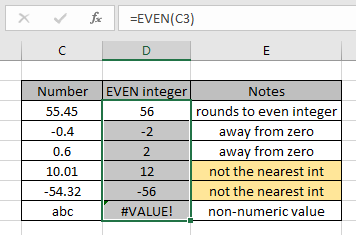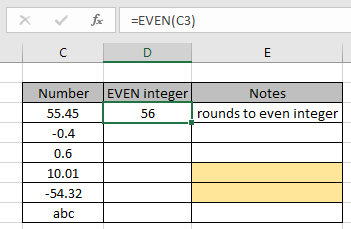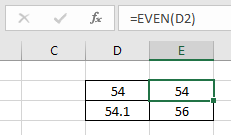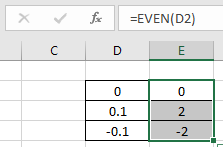# How to use the EVEN function in ExcelIn this article, we will learn how to use EVEN Function in Excel. EVEN function is a mathematical function which rounds off the given number to the nearest next even integer . EVEN function takes a number as argument and returns an even number in the cell.

Syntax:

=EVEN(number)

number : a valid number. Can be decimal

Example:

All of these might be confusing to understand. So, let's test this formula via running it on the example shown below.

Here we have some numbers to test the EVEN function in Excel.

Use the formula:

=EVEN(C3)

Explanation:As you can see the nearest next even integer to the 55.45 is 56. Here we know the nearest integer is 55 but the EVEN function returns an even integer. Now to apply the formula to the remaining cell just copy the formula using Ctrl + D taking the first cell till the last cell needed cell formula.As you can see, the formula returns only an even number using the EVEN function.

EVEN FUNCTION
Here we have one important aspect using the EVEN function in excel.
If the input number is an even number itself then the formula returns the same number but if its decimal number is near to even number then the formula doesn't return the same number. See the below image for more clarification.As you can see the formula doesn't return 54 from the given 54.1 number as input. The same result happens for the 0 number.Here are some observational notes shown below.

Notes:

1. The formula only works with numbers.
2. The formula returns the nearest next even integer given input any decimal number.
3. The function returns the number away from zero.
4. No argument to the function returns 0 as result, so do check all the blank cells.
5. The function returns #VALUE! Error if the number value is non-numeric.

Hope this article about How to use the EVEN function in Excel is explanatory. Find more articles on mathematical formulas here. If you liked our blogs, share it with your fristarts on Facebook. And also you can follow us on Twitter and Facebook. We would love to hear from you, do let us know how we can improve, complement or innovate our work and make it better for you. Write to us at info@exceltip.com.

Related Articles

How to use the ROUND function in Excel | rounds off the given number to the nearest num_digit decimal using the ROUND function in Excel

How to use the ROUNDUP function in Excel | rounds up the given number to the nearest num_digit decimal using the ROUNDUP function in Excel

How to use the ROUNDDOWN function in Excel | rounds down the given number to the nearest num_digit decimal using the ROUNDDOWN function in Excel

How to use the ISEVEN function in Excel | returns the TRUE logic value if the number is even using the ISEVEN function in Excel.

How to use the ISODD function in Excel | returns the TRUE logic value if the number is ODD using the ISODD function in Excel.

Popular Articles:

50 Excel Shortcuts to Increase Your Productivity | Get faster at your task. These 50 shortcuts will make you work even faster on Excel.

The VLOOKUP Function in Excel | This is one of the most used and popular functions of excel that is used to lookup value from different ranges and sheets.

COUNTIF in Excel 2016 | Count values with conditions using this amazing function. You don't need to filter your data to count specific values. Countif function is essential to prepare your dashboard.

How to Use SUMIF Function in Excel | This is another dashboard essential function. This helps you sum up values on specific conditions.

Terms and Conditions of use

The applications/code on this site are distributed as is and without warranties or liability. In no event shall the owner of the copyrights, or the authors of the applications/code be liable for any loss of profit, any problems or any damage resulting from the use or evaluation of the applications/code.